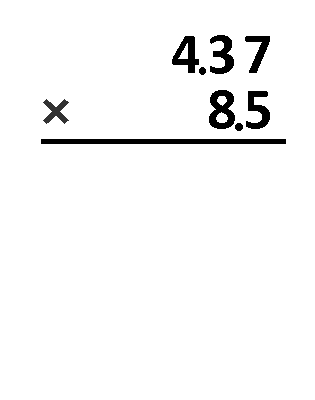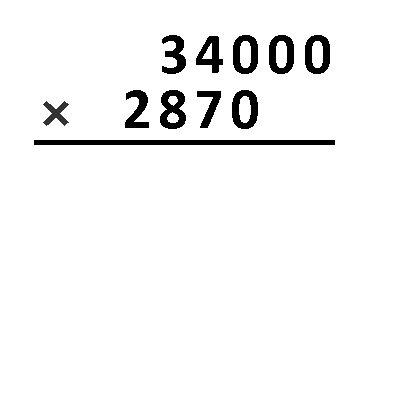# Long Multiplication Calculator

Created by Wojciech Sas, PhD
Reviewed by Steven Wooding
Last updated: Feb 02, 2023

Welcome to the long multiplication calculator - a great tool that helps you solve multiplication yourself. If you ever asked yourself: How do you multiply decimals? or How to multiply big numbers?, here is the right place to find the answer.

Knowledge of the basic multiplication algorithm makes it possible to deal with more complex problems like multiplying fractions or matrices. Furthermore, if you learn how to do long multiplication and also practice with long division calculator, it will make math exercises with operations on numbers as simple as never before!

If you'd like to try an alternative approach to multiplying large numbers, check out Omni's partial products calculator!

## How do you multiply decimals?

Let's begin from the basics - multiplication is a compact way of writing the addition of repeating numbers. If we want to solve a problem like 6×2, it would be the same as if we have to add 2 six times, 2+2+2+2+2+2. In that meaning, multiplication is extremely useful, especially for big numbers.

Mathematicians call the first number in the multiplication the multiplier and the second one the multiplicand. The result of the multiplication is the product.

💡 Multiplication is commutative. It means that we can change the multiplier and multiplicand places, and the outcome wouldn't change.

From the beginning of our education, we all learn how to multiply numbers from one to ten - most pupils' nightmare, isn't it? But, actually, it's all you need to know about how to multiply big numbers or how long multiplication with decimals works!

So, how do you multiply decimals? In short, forget about the decimal dot and perform the multiplication with whole numbers. Next, add up the decimal places in the multiplier and the multiplicand. The sum is the number of decimal places in the product. We describe the whole process in detail in the dedicated section below.

## How to do long multiplication?

We can describe the long multiplication algorithm in a few steps:

1. Set both numbers one under the other and align them to the right so that the first significant digits are the first ones from the right.

🙋 It's a good practice to set the longer number as the multiplier and the shorter one as the multiplicand. It doesn't change the product, but it does reduce the number of steps.

1. Start multiplying the multiplier by the first digit (from the right) of the multiplicand, digit by digit. Anytime you finish with a number larger than 9, write down the digit of ones and carry the digit of tens to the next step (e.g. 7×5=35, so write 5 and carry 3).

2. Repeat for the rest of the digits of the multiplier. Whenever you carry a number, add it to the product (e.g. 1×5 and 3 carried from the previous step gives us 8).

3. When you finish with the first digit of the multiplicand, you obtain a first intermediate product.

4. Repeat the same procedure for the rest of the digits from the multiplicand, each time starting one place further from the right (as you multiply by tens, hundreds, and so on). You can also write trailing zeros if you like.

🙋 If you face any 0 digits in the multiplicand, you can skip the step, as the product of zero and any other number is always zero.

1. When you're done with all of the intermediate products, add them up.

2. The result is your final product. Now you know how to do long multiplication!

## Long multiplication with decimals

Let's step up to the next level and find out how to multiply decimals using the long multiplication method. As for an example, let's multiply 4.37 by 8.5. It turns out that we can treat it as a 3- and 2-digit multiplication problem. To get the answer, we can follow these steps:1. Count the number of decimal digits in both numbers. There are two decimals in the first one and a single decimal in the second one.

2. The sum of decimal digits in the multiplier and the multiplicand is three (2+1). We will have three decimals in the product as well.

3. At this point, we can forget about decimal dots and perform the multiplication 437 × 85.

4. The product of 437 and 5 is 2185.

5. The product of 437 and 8 is 3496. Remember to start writing it one place further from the right. Alternatively, you can add one 0 at the end, so the product becomes 34960, and both numbers are right-aligned then.

6. Evaluate the sum of these two intermediate products. 2185 + 34960 = 37145.

7. Finally, apply the decimal dot in the product. We know there should be three decimal digits, so our outcome equals 37.145.

If you have any doubts, get lost at some point, or simply want to check the answer, you can always use our long multiplication calculator!

## How to multiply big numbers? Multiplication algorithm in practice

The good thing about long multiplication is that it doesn't make a problem significantly more difficult for larger numbers. What matters is the length of the numbers rather than the values themselves. Moreover, it may be even simpler to multiply big numbers if any of them (or both) ends with several trailing zeros. Why?

We can simply skip trailing zeros for multiplication, as any intermediate products would equal zero. We can add trailing zeros from both the multiplier and the multiplicand and write them next to the product. The procedure is very similar to the one with decimals.

Let's apply the long multiplication algorithm for two big numbers, let's say 34000 and 2870:1. Count the number of trailing zeros in both cases. There are three of them in the first number and a single zero in the second one.

2. Now, our new values are 34 and 287, respectively. Note that in this case, the first number is shorter than the second one (in contrast to the initial numbers). We can swap them and work out the multiplication 287 times 34.

3. The first intermediate product is 1148, and the second one is 861 (remember about shifting this number one digit to the left). Summing them up, we receive 9758.

4. That's the right time to apply missing trailing zeros to the product. We have four of them in total.

5. The final result of long multiplication is 97,580,000. We can even write it as 9.758×10⁷ using scientific notation.

## How (and when) to use the long multiplication calculator?

Have you ever tried to add or subtract fractions? If so, you're probably familiar with the concept of finding the least common denominator. Simply speaking, it's all about finding the least common multiple of two (or multiple) numbers. The process involves a certain skill in multiplication. This situation is where our long multiplication calculator becomes handy, especially for fractions containing decimals or big numbers.

The good thing is that our tool is pretty straightforward to use. As you already know how to do long multiplication with decimals by hand from previous sections, let's see how to do the same using the long multiplication calculator:

• Type the first number as the multiplier, e.g., 0.00367.

• Input the second number as the multiplicand, e.g., 449300.

• And that's all! As a result, you receive an answer, which is 1648.931. Additionally, you also get explanations and hints on how to deal with multiplying big numbers and decimals.

If you like this long multiplication calculator, make sure to check our distributive property calculator as well!

Wojciech Sas, PhD
Input two numbers
1ˢᵗ number (Multiplier)
2ⁿᵈ number (Multiplicand)
Long result
People also viewed…

### Coterminal angle

With this coterminal angle calculator you'll find some positive and negative coterminal angles, as well as the coterminal angle in the 0-360° (0-2π) range. Moreover, this tool is useful for checking if two angles are coterminal.

### Flat vs. round Earth

Omni's not-flat Earth calculator helps you perform three experiments that prove the world is round.

### Sunbathing

Do you always remember to put on sunscreen before going outside? Are you sure that you use enough? The Sunbathing Calculator ☀ will tell you when's the time to go back under an umbrella not to suffer from a sunburn!

### Union and intersection

The union and intersection calculator is here to find the union and intersection of up to three sets: either in interval notation or by inputting individual elements.